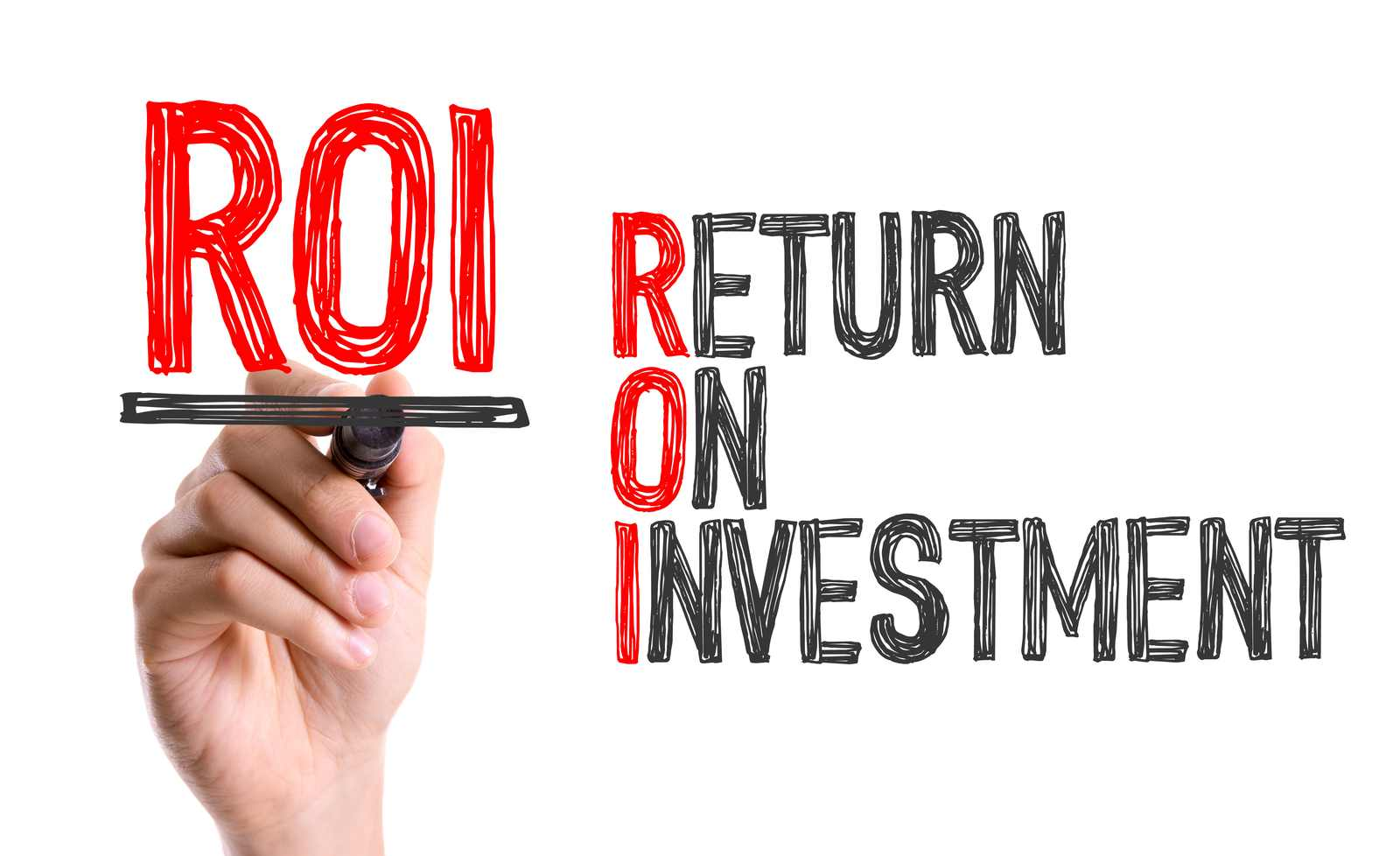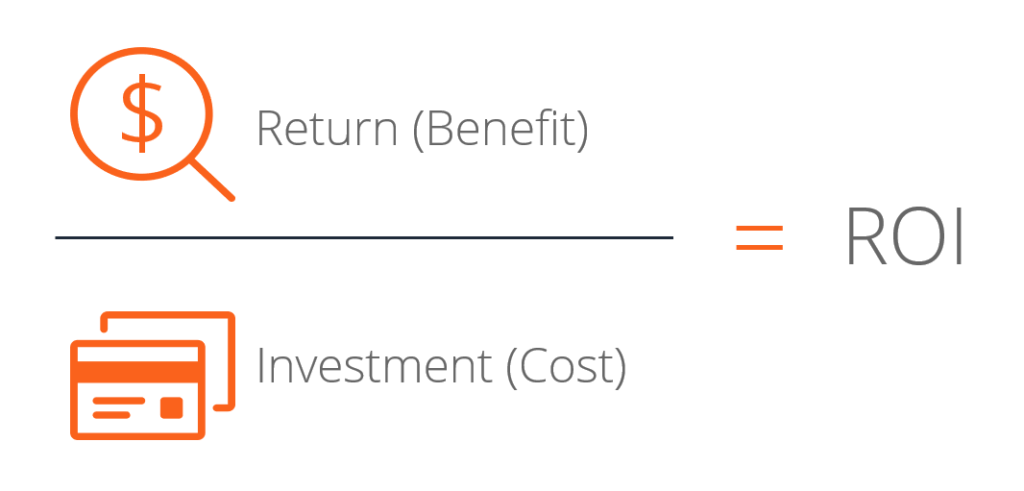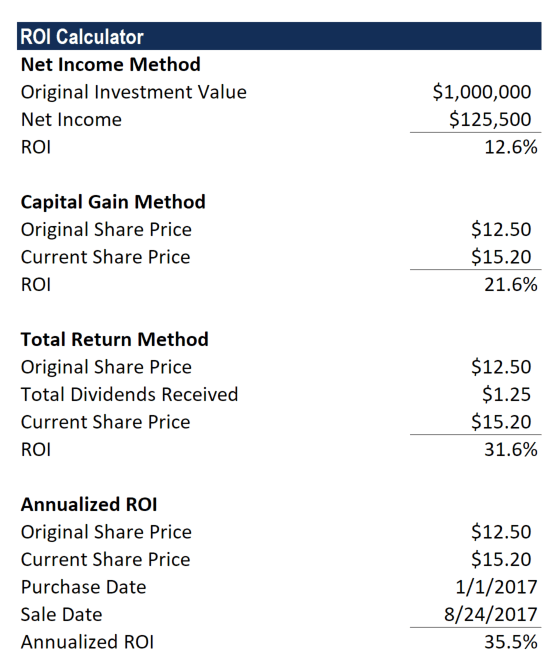# ROI Formula (Return on Investment)

Learn the different ways to calculate Return on Investment

## What is Return on Investment (ROI)?

Return on investment (ROI) is a financial ratio used to calculate the benefit an investor will receive in relation to their investment cost. It is most commonly measured as net income divided by the original capital cost of the investment. The higher the ratio, the greater the benefit earned. This guide will break down the ROI formula, outline several examples of how to calculate it, and provide an ROI formula investment calculator to download.### ROI Formula

There are several versions of the ROI formula. The two most commonly used are shown below:

ROI = Net Income / Cost of Investment

or

ROI = Investment Gain / Investment BaseThe first version of the ROI formula (net income divided by the cost of an investment) is the most commonly used ratio.

The simplest way to think about the ROI formula is taking some type of “benefit” and dividing it by the “cost”.  When someone says something has a good or bad ROI, it’s important to ask them to clarify exactly how they measure it.

### Example of the ROI Formula Calculation

An investor purchases property A, which is valued at \$500,000. Two years later, the investor sells the property for \$1,000,000.

We use the investment gain formula in this case.

ROI = (1,000,000 – 500,000) / (500,000) = 1 or 100%

### The Use of the ROI Formula Calculation

ROI calculations are simple and help an investor decide whether to take or skip an investment opportunity. The calculation can also be an indication of how an investment has performed to date. When an investment shows a positive or negative ROI, it can be an important indication to the investor about the value of their investment.

Using an ROI formula, an investor can separate low-performing investments from high-performing investments.  With this approach, investors and portfolio managers can attempt to optimize their investments.

### Benefits of the ROI Formula

There are many benefits to using the return on investment ratio that every analyst should be aware of.

##### #1 Simple and Easy to Calculate

The return on investment metric is frequently used because it’s so easy to calculate.  Only two figures are required – the benefit and the cost.  Because a “return” can mean different things to different people, the ROI formula is easy to use, as there is not a strict definition of “return”.

##### #2 Universally Understood

Return on investment is a universally understood concept so it’s almost guaranteed that if you use the metric in conversation, then people will know what you’re talking about.

### Limitations of the ROI Formula

While the ratio is often very useful, there are also some limitations to the ROI formula that are important to know.  Below are two key points that are worthy of note.

##### #1 The ROI Formula Disregards the Factor of Time

A higher ROI number does not always mean a better investment option. For example, two investments have the same ROI of 50%. However, the first investment is completed in three years, while the second investment needs five years to produce the same yield. The same ROI for both investments blurred the bigger picture, but when the factor of time was added, the investor easily sees the better option.

The investor needs to compare two instruments under the same time period and same circumstances.

##### #2 The ROI Formula is Susceptible to Manipulation

An ROI calculation will differ between two people depending on what ROI formula is used in the calculation. A marketing manager can use the property calculation explained in the example section without accounting for additional costs such as maintenance costs, property taxes, sales fees, stamp duties, and legal costs.

An investor needs to look at the true ROI, which accounts for all possible costs incurred when each investment increases in value.

### Annualized ROI Formula

As mentioned above, one of the drawbacks of the traditional return on investment metric is that it doesn’t take into account time periods. For example, a return of 25% over 5 years is expressed the same as a return of 25% over 5 days. But obviously, a return of 25% in 5 days is much better than 5 years!

To overcome this issue we can calculate an annualized ROI formula.

ROI Formula:
= [(Ending Value / Beginning Value) ^ (1 / # of Years)] – 1

Where:

# of years = (Ending date – Starting Date) /  365

For example, an investor buys a stock on January 1st, 2017 for \$12.50 and sells it on August 24, 2017 for \$15.20. What is the regular and annualized return on investment?

Regular = (\$15.20 – \$12.50) / \$12.50 = 21.6%

Annualized = [(\$15.20 / \$12.50) ^ (1 / ((Aug 24 – Jan 1)/365) )] -1 = 35.5%

### ROI Formula Calculator in Excel

Download CFI’s free ROI Formula Calculator in Excel to perform your own analysis.  The calculator uses the examples explained above and is designed so that you can easily input your own numbers and see what the output is under different scenarios.

The calculator covers four different ROI formula methods: net income, capital gain, total return, and annualized return.The best way to learn the difference between each of the four approaches is to input different numbers and scenarios, and see what happens to the results.

### Video Explanation of Return on Investment/ROI Formula

Below is a video explanation of what return on investment is, how to calculate it, and why it matters. You’ll learn a lot in just a couple of minutes!

### Alternatives to the ROI Formula

There are many alternatives to the very generic return on investment ratio.

The most detailed measure of return is known as the Internal Rate of Return (IRR). This is a measure of all the cash flow received over the life of an investment, expressed as an annual percentage (%) growth rate. This metric takes into account the timing of cash flow, which is a preferred measure of return in sophisticated industries like private equity and venture capital.

Other alternatives to ROI include Return on Equity (ROE) and Return on Assets (ROA).  These two ratios don’t take into account the timing of cash flows and represent only an annual rate of return (as opposed to a lifetime rate of return like IRR). However, they are more specific than the generic return on investment since the denominator is more clearly specified.  Equity and Assets have a specific meaning, while “investment” can mean different things.

Thank you for reading this CFI guide to calculating return on investment. CFI is the official global provider of the Financial Modeling Analyst designation. To continue learning and advancing your career, these additional resources on rates of return will be helpful:

• Return on Equity (ROE)
• Return on Assets (ROA)
• Internal Rate of Return (IRR)
• DCF Modeling

### Financial Analyst Training

Get world-class financial training with CFI’s online certified financial analyst training program!

Gain the confidence you need to move up the ladder in a high powered corporate finance career path.

Learn financial modeling and valuation in Excel the easy way, with step-by-step training.